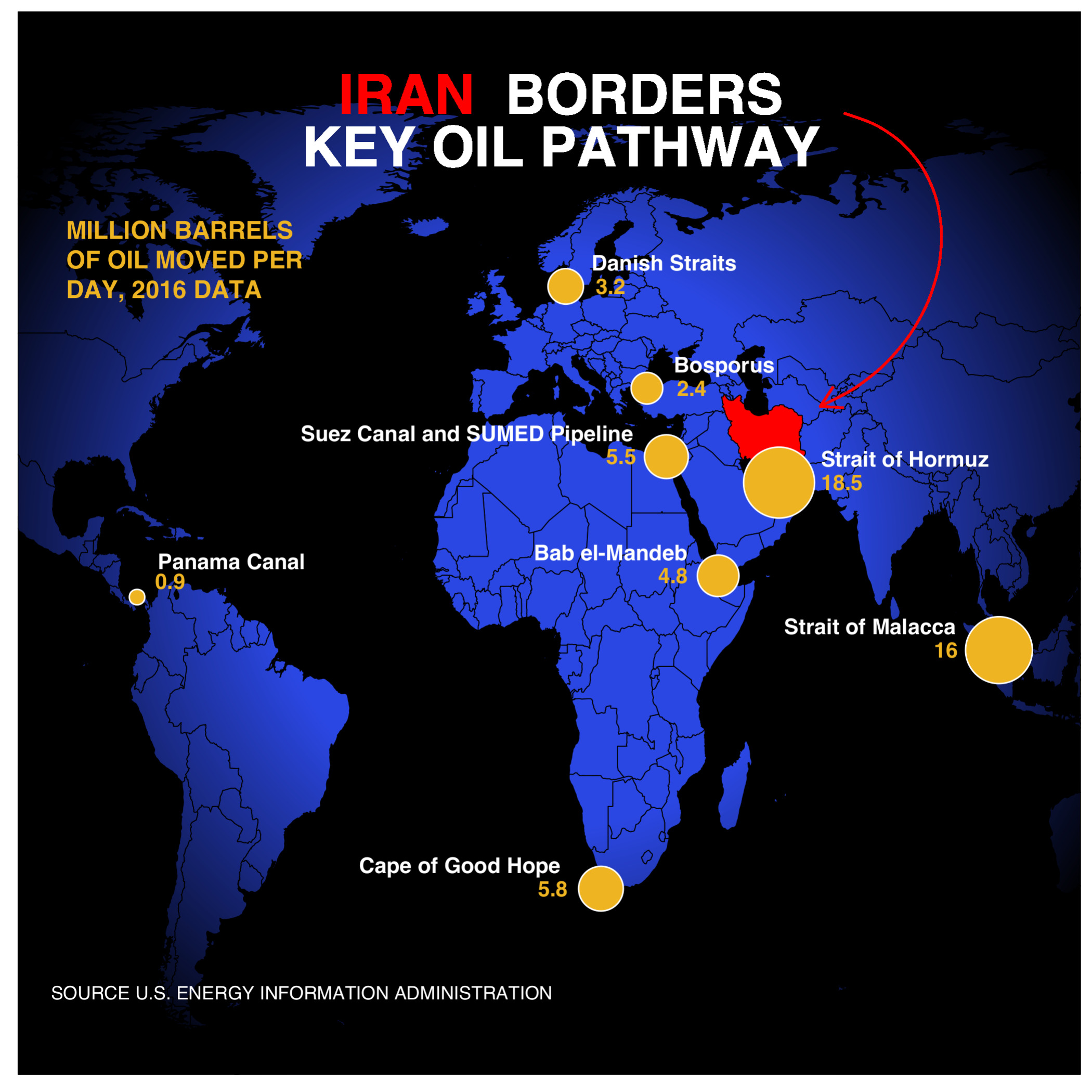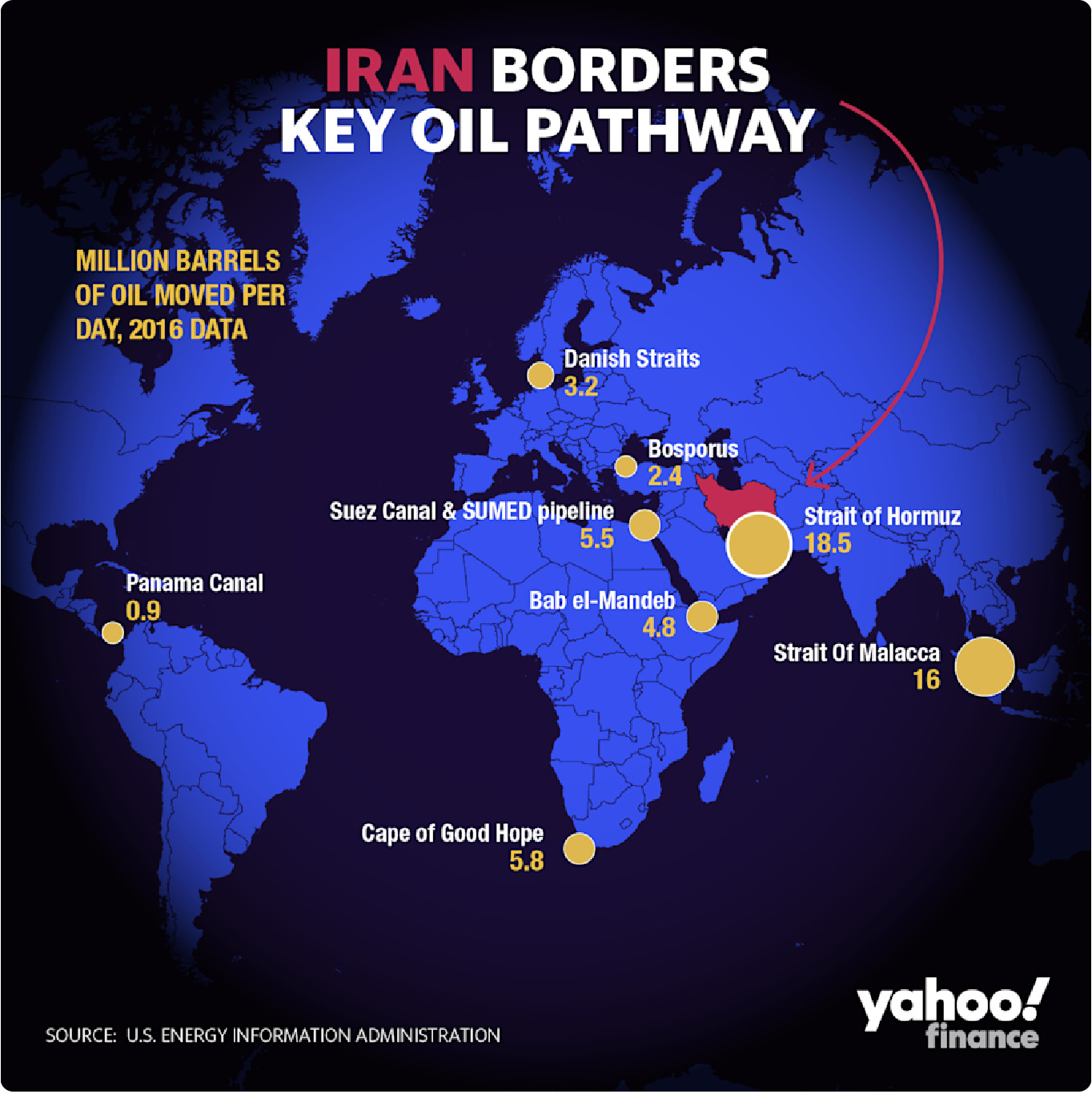R package build · 2021/10/06 · 3 minute read

### Intro

Early last year I posted on my blog an attempt to recreate a Yahoo Finance graphic. Now, thanks to the work of Dr Paul Murrell on the grid and gggrid packages, I have the tools to polish this one off. Reference Article

Here is a streamlined version of my earlier post, and the graphic enhanced by the radial gradient feature.

### My attempt to replicate### The original fileSource Article

### Required Packages

library(tidyverse)
library(sf)
library(rnaturalearth)
library(rnaturalearthdata)
library(ggtext)
library(grid)
library(gggrid)

### The Data

Will cut right to the chase this time. Here are the data elements we need for the map and the points on the map.

world <- ne_countries(scale = "medium", returnclass = "sf")
data <- data.frame(Location = c("Strait of Hormuz", "Strait of Malacca",
"Suez Canal and SUMED Pipeline", "Bab el-Mandeb", "Danish Straits",
"Bosporus", "Panama Canal", "Cape of Good Hope"),
Barrels = c(18.5,16, 5.5, 4.8, 3.2, 2.4, 0.9, 5.8),
Lat = c(26.5667, 1.43, 30.455, 12.5833, 56, 40.7225, 9.38743, -34.3548),
Lon = c(56.25, 102.89, 32.35, 43.3333, 11, 28.2247, -79.91863, 18.4698))

### The Graph

Note the limitations on the graphic devices that can be used. Here I will use cairo_ps to save the output locally as a postscript file. The additions to achieve the radial gradient are grid::radialGradient() and gggrid::grid_panel().

# Not Run
#cairo_ps(filename  = "plot2.ps")
#title <- "<b style='color:#FF0000'>IRAN</b> <b style='color:#FFFFFF'>BORDERS<br> KEY OIL PATHWAY</b>"
#ggplot(data = world) +
#    geom_sf(fill = "#2B47E3", color = "black", size = 0.2) +

#    geom_sf(data = subset(world, geounit == "Iran"), fill = "red", color = "black", size = 0.2) +
#    geom_point(data = data, aes(x =Lon, y=Lat, size = Barrels),
#               shape = 21, fill = "goldenrod2", color = "white", show.legend = F) +
#    geom_text(data = data, aes(x =Lon, y=Lat, label = Location), color = "white", size = 3.5,
#              vjust = c(-1, -1, -1, -1, -1, -1, -1.8, -1), hjust = c(-0.25, 1.25, 1.1, 1.2, -0.18, -0.27, -0.14, 1.2),
#              fontface = "bold") +
#    geom_text(data = data, aes(x =Lon, y=Lat, label = Barrels), color = "goldenrod2", size = 3.5,
#              vjust = c(.5, .5, .5, .5, .5, .5, -.5, .5), hjust = c(-1.0, 2.7, 2.0, 2.0, -1.0, -1.0, -0.6, 2.1),
#              fontface = "bold") +
#    scale_size_continuous(range = c(3, 15)) +
#    annotate("text", x = -95, y = 60, label = "MILLION BARRELS\nOF OIL MOVED PER\nDAY, 2016 DATA", size = 4.0,
#             color = "goldenrod2", fontface= "bold", hjust = 0, lineheight = 1) +
#    annotate("text", x = -45, y = -50, label = "SOURCE U.S. ENERGY INFORMATION ADMINISTRATION", size = 3.0,
#             color = "white")+
#    geom_richtext(x = 10, y = 80, label = title, size = 9, fill = NA, label.color = NA, lineheight = 0.33) +
#    geom_curve(aes(x = 70, y = 82, xend = 65, yend = 38), color = "red", arrow = arrow(type = "open",
#              length = unit(0.15, "inches")), curvature = -0.75, angle = 100, ncp =10) +
#    theme(panel.background = element_rect(fill = "black"),
#          panel.grid = element_blank(), axis.title = element_blank(), axis.ticks = element_blank(),
#          axis.text = element_blank(), aspect.ratio = 1) +
#    xlim(-95.00, 110.00) +
#    ylim(-55, 90)
#dev.off()